## Topic 6.3: Transformations to Linear Regression (Matlab)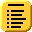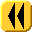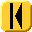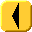Introduction Notes Theory HOWTO Examples Engineering Error Questions Matlab Maple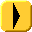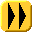Finding the coefficient vector in Matlab is very simple:

```x = [0.53, 0.75, 1.22, 2.11, 3.25]';
y = [0.78, 0.81, 0.97, 1.28, 1.82]';
V = [x, ones(size(x))];
c = V \ log(y);
```

To plot the points and the best fitting curve, you can enter:

```xs = (0:0.1:4)';
plot( x, y )
hold on
plot( xs, exp(c(1)*xs + c(2)) );
```

Be sure to issue the command hold off if you want to start with a clean plot window.